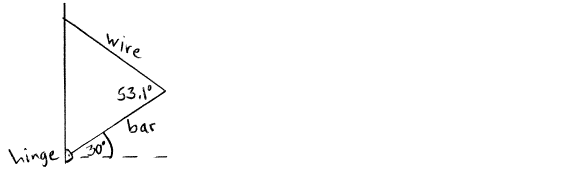Problem: A uniform bar (I = 1/3 ML2 for an axis at one end) has mass M = 8.00 kg and length L = 6.00 m. The lower end of the bar is attached to a wall by a frictionless hinge. The bar is held at an angle of 30.0° above the horizontal by a wire that is attached between the upper end of the bar and the wall. The wire makes an angle of 53.1° with the bar. If the wire breaks, what is the initial acceleration (in rad/s 2) of the bar, just after the wire breaks?

94% (2 ratings)
Problem Details

A uniform bar (I = 1/3 ML2 for an axis at one end) has mass M = 8.00 kg and length L = 6.00 m. The lower end of the bar is attached to a wall by a frictionless hinge. The bar is held at an angle of 30.0° above the horizontal by a wire that is attached between the upper end of the bar and the wall. The wire makes an angle of 53.1° with the bar. If the wire breaks, what is the initial acceleration (in rad/s 2) of the bar, just after the wire breaks?